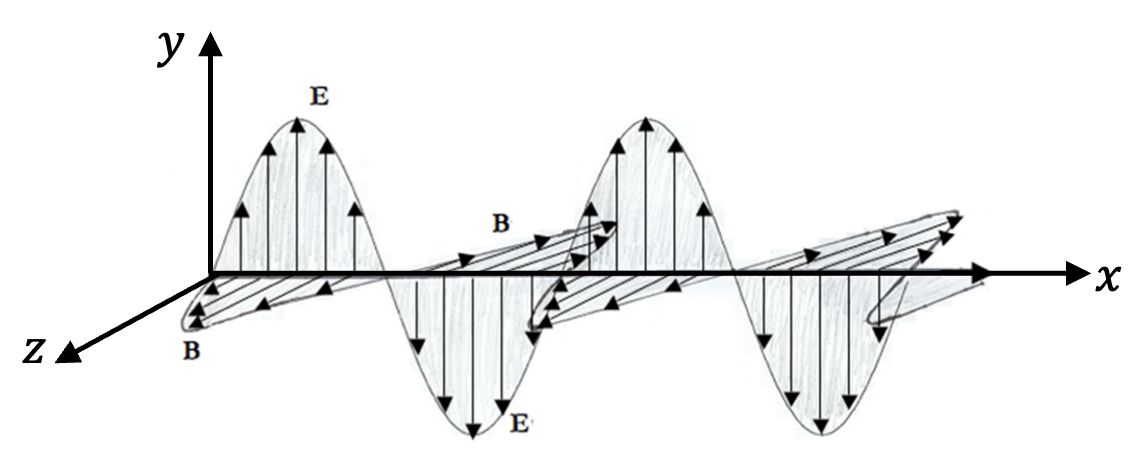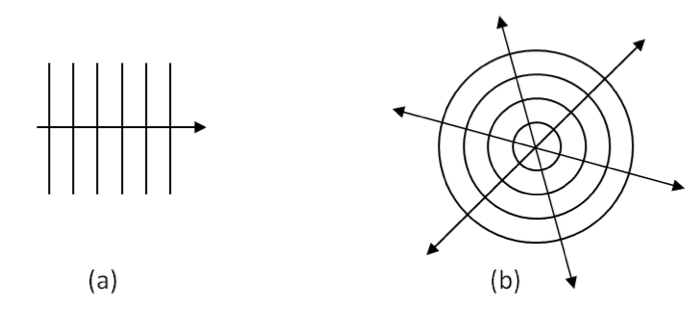## Section49.1Electromagnetic Wave

The wave nature of light is essential for many phenomena exhibited by light. In 1865, James Clerk Maxwell identified light as intertwined waves of electric and magnetic fields. These waves are called electromagnetic waves. Before Maxwell, optics and electromagnetism were two separate branches of study in physics. Maxwell showed that optics is actually a subfield of electromagnetism.

According to the Maxwell's equations of electricity and magnetism, an oscillating electric field induces an oscillating magnetic field, which in turn induces oscillating electric field. This explains how light can travel in vacuum without needing any material medium. Maxwell also showed that the electric and magnetic fields of an electromagnetic wave in vacuum are perpendicular to each other, and they in turn are perpendicular to the direction of the propagation of the wave.

A particularly simple form of electromagnetic wave is a plane harmonic wave in which electric field oscillates along a single axis, and magnetic field in an axis perpendicular to that as shown in Figure 49.1.1. For instance, an electromagnetic wave traveling towards positive $x$ axis with electric field oscillating along $y$ axis and magnetic field oscillating $z$ axis is an example of a plane wave.Figure 49.1.1. Plane electromagnetic wave traveling to the right. The electric field and magnetic field are perpendicular to each other and direction of the wave is perpendicular to both electric and magnetic fields.

We can write the plane electromagnetic wave shown in Figure 49.1.1 analytically by two vector functions, one the electric field and the other for the magnetic field. The two waves have the same angular frequency $\omega\text{,}$ same wavenumber $k$ , and same phase constant $\phi\text{.}$ Let us denote their amplitudes by $E_0$ and $B_0\text{,}$ respectively. With unit vectors $\hat u_y$ towards positive $y$ axis and $\hat u_z$ towards positive $z$ axis, the following functions will describe oscillating electric and magnetic fields propagating towards positive $x$ axis.

\begin{align} \amp \vec E = E_y \hat u_y = \hat u_y E_0 \cos(k x -\omega t + \phi),\label{eq-em-wave-1}\tag{49.1.1}\\ \amp \vec B = B_z \hat u_z = \hat u_z B_0 \cos(k x -\omega t + \phi).\label{eq-em-wave-2}\tag{49.1.2} \end{align}

The amplitudes of the waves, viz. $E_0$ and $B_0$ are related by the wave speed $v$ in the medium. We will use symbol $c$ for the speed in vacuum.

\begin{equation} B_0 = \frac{E_0}{v}.\tag{49.1.3} \end{equation}

With $\hat u_x$ as unit vector towards positive $x$ axis, the direction of the wave is given by the wave propagation vector, $\vec k = k \hat u_x\text{,}$ which is the product of the wavenumber and the direction unit vector.

The argument of the trigonometric functions on the right of Eqs. (49.1.1) and (49.1.2) is called the phase of the wave. As shown in these formulas, the waves of these fields march with the same phase: the crest of the electric field occurs at the same location and time as the crest of the magnetic field wave and the trough with the trough.

The angular frequency $\omega$ (radians per second) and wave number $k$ (radians per meter) are related to the frequency $f$ (cycles per second or Hertz, Hz) and wavelength $\lambda$ (meter) respectively.

\begin{align*} \amp \omega = 2\pi f.\\ \amp k = \frac{2\pi}{\lambda}. \end{align*}

The ratio $\omega/k$ is therefore equal to the wavespeed $v$ in the medium by the following fundamental equation of waves.

\begin{equation} \frac{\omega}{k} = \lambda f = v.\tag{49.1.4} \end{equation}

Maxwell showed that the speed of electromagnetic wave in the vacuum is simply related to the electrromagnetic properties of vacuume, namely, electric susceptibility $\epsilon_0$ and the magnetic permeability $\mu_0\text{.}$

\begin{equation} \text{Speed of light in vacuum, }\ \ c = \frac{1}{\sqrt{\mu_0 \epsilon_0}}.\label{eq-speed-of-light-in-vaccum-ch-light-wave}\tag{49.1.5} \end{equation}

In other linear media, a similar formula relates speed to electric susceptibility $\epsilon$ and the magnetic permeability $\mu$ of the medium.

\begin{equation} v = \frac{1}{\sqrt{\mu\epsilon}}.\tag{49.1.6} \end{equation}

It turns out that, for nonmagnetic media, $\mu\approx \mu_0\text{.}$ Therefore,

\begin{equation} v = \sqrt{\frac{\epsilon}{\epsilon_0}} \; c.\tag{49.1.7} \end{equation}

The ratio $\frac{\epsilon}{\epsilon_0}$ is called the dieelectric constant of the medium, which is denoted by $\epsilon_r\text{.}$

\begin{equation} v = \sqrt{\epsilon_r} \; c.\tag{49.1.8} \end{equation}

Refractive index $n$ of the medium is related to the dielectric constant by

\begin{equation} n = \frac{1}{\sqrt{\epsilon_r}}.\tag{49.1.9} \end{equation}

Therefore, $v$ in a medium can also be written as

\begin{equation} v = \frac{c}{n}.\tag{49.1.10} \end{equation}

Since $\epsilon_0$ and $\mu_0$ are universal values, speed of light in vacuum, giben by Eq. (49.1.5), has the following exact alue.

\begin{equation} c = 299,792,458\ \text{m/s}\ \ \ \text{(Exact)}\tag{49.1.11} \end{equation}

Since electric and magnetic fields of an electromagnetic wave are interdependent, it often suffices to examine only the electric field of the wave. You should, however, keep in mind that magnetic field wave is also present.

The points in space with equal phase form a surface called the wavefront. Evidently, wave function has the same value at all points of the wavefront. We see that the wavefronts of the plane waves given in Eqs. (49.1.1) and (49.1.2) are planes perpendicular to the direction of the wave. That is why these waves are also called plane waves.

Let us examine the crest of the waves at $t=0\text{.}$ The crests will occur when the argument of the cosine is equal to $2n\pi$ for $n$ an integer.

\begin{equation} -kx + \phi = 2 n \pi\ \ \Longrightarrow\ \ x = \text{constant}\tag{49.1.12} \end{equation}

Thus, a plane wave moving along $x$ axis crests simultaneously at all points of planes parallel to the $yz$ plane separated by one wavelength.

Not all waves are plane waves. For instance, an isotropic light emitted by a point source will spread out as spherical wave fronts centered at the source. These waves are called spherical waves. The traveling spherical waves are given by a wave function whose amplitude drops off inversely with the distance $r$ from the source. With $\hat u_r$ a unit vector pointed radially outward from the origin, we get the following for a spherical wave.

\begin{equation} \vec E = \hat u_r \frac{V_0}{r}\ \cos(kr - \omega t + \phi),\tag{49.1.13} \end{equation}

where $V_0$ is a constant amplitude with units of electric potential. Pictorially, a traveling electromagnetic wave of a single frequency can be represented by drawing wavefronts that are a single wavelength apart. Rather than draw a three-dimensional plot it is customary to draw a cross-section. Thus, for a plane wave we draw parallel lines, each line representing a plane wavefront, while for a spherical wave we draw arcs or circles, each representing a spherical wavefront (Figure 49.1.2). Note that the wave fronts are perpendicular to the direction of wave.Figure 49.1.2. Wave fronts are perpendicular to the waves: (a) plane wave, (b) spherical wave.

The electric field of an electromagnetic wave in vacuum is given by the following vector function of space and time, where $y$ is in meters and $t$ is in seconds.

\begin{equation*} \vec E = \hat u_x\ 3\times 10^{5}(\text{N/C})\ \cos\left(2\pi\times 10^{15}\ t + k y + \frac{\pi}{2} \right), \end{equation*}

where $\hat u_x$ is a unit vector pointed towards positive $x$ axis.

(a) Find the values of the frequency, wavenumber and wavelength of the wave.

(b) What is the direction of the travel of the wave? Find the propagation vector for the wave.

(c) Find the associated magnetic field wave.

Hint

See the discussion in this section.

(a) $f=10^{15}\text{ Hz}\text{,}$ $k=2.09\times 10^{7}\text{ m}^{-1}\text{,}$ $\lambda=3\times 10^{-7}\text{ m}\text{.}$ (b) $\vec k = - 2.09\times 10^{7}\text{ m}^{-1} \hat u_y\text{.}$ (c) $\vec B = \hat u_z 10^{-3}\text{ T} \cos\left( \pi\times 10^{15}\ t + 2.09\times 10^{7} y + \frac{\pi}{2} \right) \text{.}$

Solution 1 (a)

(a) From the phase part, i.e., the argument of the cosine, we read off the number multiplying time to get the angular frequency, $\omega\text{.}$

\begin{equation*} \omega = 2\pi\times 10^{15}\text{ sec}^{-1}. \end{equation*}

Dividing this by $2\pi$ will give the cycles per sec or $\text{Hz}\text{.}$

\begin{equation*} f = \frac{\omega}{2\pi} = 10^{15}\text{ Hz}. \end{equation*}

Similarly, we readoff the wavenumber from the number that is multiplying the space, here $y\text{.}$ We see that it is $k\text{.}$ So, we need to think of another way of getting numerical value of $k\text{.}$ We know that the product of frequency and wavelength is speed, we know for EM wave in vacuum. In terms of angular quantities this becomes

\begin{equation*} \frac{\omega}{k} = c. \end{equation*}

Therefore,

\begin{equation*} k = \frac{\omega}{c} = \frac{2\pi\times 10^{15}\text{ sec}^{-1}}{3\times 10^8\text{ m/s}} = 2.09\times 10^{7}\text{ m}^{-1}. \end{equation*}

We get wavelength $\lambda$ from $k$ by

\begin{equation*} \lambda = \frac{2\pi}{k} = 3\times 10^{-7}\text{ m}. \end{equation*}
Solution 2 (b)

(b) By setting the argument of cosine to zero, we see that $y/t \lt 0\text{.}$ This means the wave is traveling towards negative $y$ axis. The popagation vector is product of the wavenumber and a unit vector along the direction of wave. Therefore,

\begin{equation*} \vec k = - 2.09\times 10^{7}\text{ m}^{-1} \hat u_y, \end{equation*}

where I have used the symbol $\hat u_y$ for the unit vector in the direction of positive $y$ axis. With negative multiplying the unit vector, the direction of $\vec k$ is towards negative $y$ axis.

Solution 3 (c)

(c) We need to obtain the direction of $\vec B$ and magnitude of the amolitude $B_0\text{.}$ Lets work out the direction first. The direction of $\vec E\text{,}$ $\vec B\text{,}$ and propagation vector $\vec k$ are related for an EM wave.

\begin{equation*} \text{ Direction of }\vec k \text{ is direction of } \vec E\times \vec B\text{ product}. \end{equation*}

Now, use right hand to figure the direction of $\vec B$ given that $\vec E$ is towards positive $x$ axis and $\vec k$ is towards negative $y$ axis. I got the direction of $\vec B$ to be towards positive $z$ axis.

The magnitude of $B_0$ is $E_0/c\text{,}$ therefore

\begin{equation*} B_0 = \frac{E_0}{c} = \frac{3\times 10^{5}\text{ N/C}}{3\times 10^{8}\text{ m/s}} = 10^{-3}\text{ T}. \end{equation*}

Therefore, magnetic field wave function is

\begin{equation*} \vec B = \hat u_z 10^{-3}\text{ T} \cos\left( \pi\times 10^{15}\ t + 2.09\times 10^{7} y + \frac{\pi}{2} \right), \end{equation*}

where I hae used $\hat u_z$ for the unit vector pointed towards ppositive $z$ axis.